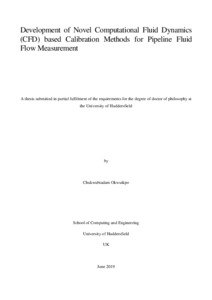# Development of Novel Computational Fluid Dynamics (CFD) based Calibration Methods for Pipeline Fluid Flow Measurement

Okwuikpo, Chukwubiadam (2019) Development of Novel Computational Fluid Dynamics (CFD) based Calibration Methods for Pipeline Fluid Flow Measurement. Doctoral thesis, University of Huddersfield.Preview
PDF - Accepted Version

## Abstract

The measurement of fluid flows is encountered in most engineering applications, such as oil and gas, power etc. Lack of efficient and straightforward methods of flow measurement cause inaccuracies in process control. One of the instruments that are widely used for measurements is the five-hole pressure probe because of its effectiveness in measuring flow parameters such as the velocity of the flow stream, static and stagnation pressures.

The present study describes the design, manufacture and Computation fluid dynamics (CFD) based novel methods of calibration of conical and hemispherical five-hole pressure probes appropriate for three-dimensional flow field measurements, capable of improving calibration and flow measurement accuracies. The conical and hemispherical five-hole pressure probes have been designed and tested in a wind tunnel and hydraulic pipeline flows respectively. The correlation between each geometrical shape of the probes and their yaw and pitch angles flow interference have been investigated. More specifically, the effect of each probe shape on the calibration of the process in the wind tunnel have been investigated. Furthermore, the effect of the hydraulic pipeline flow regime and fluid properties on the calibration process has been investigated, and comparisons have been made for results obtained in air and water flows.

The novel method of calibration is based on the use of Computational Fluid Dynamics (CFD) techniques to develop calibration methods for five-hole probes and incorporating these calibration methods into fluid flow measurements. Even though the concept of using CFD to analyse the flow around a five-hole probe numerically has been in practice for more than a decade now, there are severe limitations to it. These limitations range from the accuracy of the numerical and experimental data. In the present study, these two factors have been thoroughly investigated, leading to the accurate integration of numerical datasets into the conventional experimental calibration datasets for both wind tunnel and in hydraulic pipeline flows to cover a wide range of applications.

The first aspect of this study has been to carry out the calibration of a five-hole pressure probe using standard experimental methods, in a wind tunnel and hydraulic pipeline flows respectively. A pressure normalisation and data analysis technique introduced by Paul Akshoy coupled with sixth order regression method have been used for calibrations dataset analysis to improve the accuracy of calibration. The calibration maps, calibration coefficients and coefficients of determination have been calculated using sectoring scheme. However, due to several sources of error in several calibration procedures, the calibration coefficients of determination are not usually up to the expected accuracy level needed for accurate flow measurements. Therefore, by first carrying out the conventional calibration of the five-hole pressure probe using sectoring scheme, the coefficients of determination, also known as calibration constants (a0 to a27) are calculated. The calibration determinants, which performed less than 90%, are assumed inaccurate and are marked for improvements.

The second method of calibration developed in this study (CFD based numerical calibration method) has been to repeat the calibration of the five-hole probe using numerical datasets generated through the use of CFD simulations under the same flow and system conditions. The numerical calibration coefficients, calibration maps and coefficients of determination are calculated and tabulated. The third aspect of this study is to identify the sector of the experimental calibrations that has its coefficients of determination less than 90% assumed to be contributing a factor of measurements inaccuracies, this coefficient of calibration is replaced by the highly accurate CFD based numerical coefficients of calibration with above 90% accuracy. Hence integrating the two methodologies to develop a novel calibration method.

The process of integrating CFD based numerical datasets into experimental datasets is the second novel method of calibrations developed in this study, known as CFD based integration calibration. The same calibration technique used for the previous two methods is applied to the CFD based integrated datasets to generate calibration coefficients, calibration maps and calibration coefficients of determination and tabulated. The performances of the calibration method are tested by comparing their results for accuracy. For each wind tunnel and hydraulic pipeline flow respectively, and for the conical and hemispherical five-hole probe, the calibration procedures for all the novel based CFD calibration methods are the same.

The fourth and last aspect of the of this study has been to extend the calibration angles (pitch and yaw) of the CFD based integrated calibration method of the probes, through the use of CFD based numerical simulation. It involves using the CFD technique to simulate each probe for ±35⁰ to ± 45⁰ under their respective flow conditions, acquire the pressure datasets, and reapply the calibration formula, pressure normalisation, and data reduction technique to obtain calibration data that can be used in systems with larger flow angles to cover a wide range of space for measurements.

Item Type: Thesis (Doctoral) T Technology > TA Engineering (General). Civil engineering (General) School of Computing and Engineering Andrew Strike 12 Sep 2019 09:07 28 Aug 2021 14:50 http://eprints.hud.ac.uk/id/eprint/34990View Item